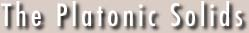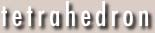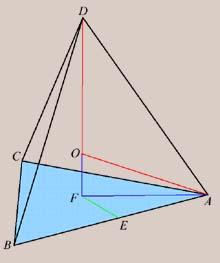Last revision Wednesday 28 August 1996Tetrahedron (f = 4, e = 6, v = 4)  An interesting excercise is to determine the exradius Rt and inradius rt of the tetrahedron in terms of the length of an edge; that is, the radii of the circumscribed and inscribed spheres, respectively. This is done with the help of basic geometry and the Pythagorean Theorem. The main observation here is to note that the lengths OA and OD are equal, since O is the center of the tetrahedron. What we wish to determine, then, are the lengths Rt = OD and rt = OF. Set the length of an edge of the tetrahedron to be 1. Then AE = 1/2. Note triangle AEF is a 30-60-90 right triangle, since angle CAB = 60 and F, being the foot of the perpendicular dropped from center O, is the center of equilateral triangle ABC. Thus AF is twice EF, and AE is Sqrt times EF. Hence EF = 1/(2 Sqrt) and AF = 1/Sqrt. Since AF2 + DF2 = AD2 = 1, DF2 = 1 - 1/3 = 2/3. Using the observation that OA = OD, we have It follows that the inradius is simply DF - OD = Sqrt[2/3] - Sqrt/4 = 1/(2 Sqrt).